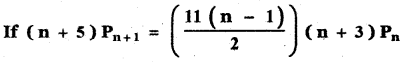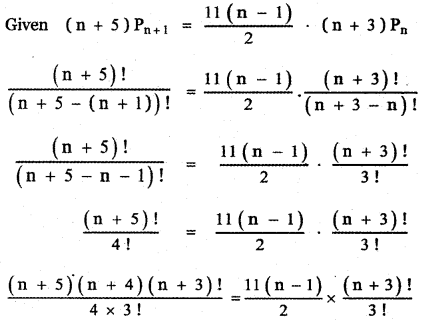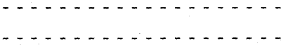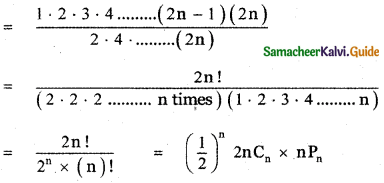Tamilnadu State Board New Syllabus Samacheer Kalvi 11th Maths Guide Pdf Chapter 4 Combinatorics and Mathematical Induction Ex 4.5 Text Book Back Questions and Answers, Notes.

## Tamilnadu Samacheer Kalvi 11th Maths Solutions Chapter 4 Combinatorics and Mathematical Induction Ex 4.5

Choose the correct or the most suitable answer:

Question 1.
The sum if the digits at the 10th place of all numbers formed with the help of 2, 4, 5, 7 taken all at a time is
(1) 432
(2) 108
(3) 36
(4) 18
(2) 108Explaination:Number of digits given = 2, 4, 5, 7
Number of 4 digit numbers formed = 4! =24
So each digit occur $$\frac{24}{4}$$ = 6 times
Sum of the digits = 2 + 4 + 5 + 7 = 18
So sum of the digits in each place = 18 × 6 = 108

Question 2.
In an examination, there are three multiple-choice questions and each question has 5 choices. The number of ways in which a student can fail to get all answer correct is
(1) 125
(2) 124
(3) 64
(4) 63
(2) 124

Explanation:
Number of multiple-choice questions = 3
Number of choices in each question = 5
Number of ways of answering = 5 × 5 × 5 = 53 = 125
Number of ways of getting all answer correct = 1
∴ Number of ways of getting incorrect answer = 125 – 1 = 124Question 3.
The number of ways in which the following prize be given to a class of 30 boys first and second in mathematics, first and second in Physics, first in Chemistry and first in English is
(1) 304 × 292
(2) 302 × 293
(3) 302 × 294
(4) 30 × 295
(1) 304 × 292

Explanation:
I and II in maths can be given in 30 × 29 ways.
I and II in physics can be given in 30 × 29 ways.
I and chemistry can be given in 30 ways.
I in English can be given in 30 ways.
So total number of ways = 30 × 29 × 30 × 29 × 30 × 30 = 304 × 292

Question 4.
The number of 5 digit numbers all digits of which are odd is
(1) 25
(2) 55
(3) 56
(4) 625
(2) 55

Explanation:
The odd digit numbers = 1, 3, 5, 7, 9
Since repetition is allowed, each digit can be filled by 1, 3, 5, 7, 9
Unit’s place can be filled in 5 ways
Ten’s place can be filled in 5 ways etc.
∴ The number of 5 digit numbers with an odd digit in each place = 5 × 5 × 5 × 5 × 5 = 55 ways

Question 5.
In 3 fingers, the number of ways four rings can be worn is ————- ways.
(1) 43 – 1
(2) 34
(3) 68
(4) 64
(4) 64

Explanation:
Each letter can be ported in 3 ways
∴ 4 letter is 34 waysQuestion 6.then the value of n are
(1) 7 and 11
(2) 6 and 7
(3) 2 and 11
(4) 2 and 6
(2) 6 and 7

Explaination:( n + 5 ) ( n + 4) = 2 × 11 × (n – 1)
n2 + 5n + 4n + 20 = 22n – 22
n2 + 9n + 20 – 22n + 22 = 0
n2 – 13n + 42 = 0
n (n – 6) – 7 (n – 6) = 0
(n – 7) (n – 6) = 0
n – 7 = 0 or n – 6 = 0
n = 7 and n = 6Question 7.
The product of r consecutive positive integers is divisible by
(1) r !
(2) (r – 1) !
(3) ( r + 1 ) !
(4) rr
(1) r !

Explanation:
1(2) (3) ….. (r) = r! which is ÷ by r!

Question 8.
The number of five-digit telephone numbers having at least one of their digits repeated is
(1) 90000
(2) 10000
(3) 30240
(4) 69760
(4) 69760

Explaination:
Case (i) When zero is allowed in the first place.
The number of five-digit telephone numbers which can
be formed using the digits 0, 1, 2, …………,9 is 105.
The number of five-digit telephone numbers which have none of their digits repeated is 10P5
= 10 × 9 × 8 × 7 × 6 = 30240
∴ The required number of telephone number
= 105 – 30240
= 100000 – 30240
= 69,760

Case (ii) When zero is not allowed in the first place,
(a) Repetition allowed:The number of ways of filling the first box, second box, …………….. , fifth box
= 9 × 10 × 10 × 10 × 10
= 90000(b) Repetition riot allowed:
Number of ways of filling 1 box = 9
Number of ways of filling 2nd box = 9
Number of ways of filling 3rd box = 8
Number of ways of filling 4th box = 7
Number of ways of filling 5th box = 6
∴ Number of numbers with no digit is repeated = 9 × 9 × 8 × 7 × 6 = 37216
∴ Number of numbers having atleast one of the digits repeated = 90000 – 37216 = 52,784

Question 9.
If (a2 – a ) C2 = ( a2 – a) C4 then the value of a is
(1) 2
(2) 3
(3) 4
(4) 5
(2) 3

Explaination:
(a2 – a)C2 = (a2 – a) C4
(a2 – a)C2 = (a2 – a) C(a2 – a) – 4 [nCr = nCn-r]
(a2 – a)C2 = (a2 – a) Ca2 – a – 4
∴ 2 = a2 – a – 4
a2 – a – 4 – 2 = 0 ⇒ a2 – a – 6 = 0
a2 – 3a + 2a – 6 = 0
a (a – 3) + 2 (a – 3) = 0
⇒ (a + 2)(a – 3) = 0 ⇒ a = – 2 or 3

Question 10.
There are 10 points in a plane and 4 of them are collinear. The number of straight lines joining any two points is
(1) 45
(2) 40
(3) 39
(4) 38
(2) 40

Explaination:
Total number of points = 10
The number of straight lines joining any two points is same as the number ways of selecting 2 points from 10 points.
∴ The number of straight lines = 10C2
Given 4 points are collinear.
∴ The number of straight lines that these 4 points contribute = 4C2
Since 4 points are collinear they lie on a line.
∴ Required number of lines = 10C2 – 4C2 + 1= 5 × 9 – 2 × 3 + 1
= 45 – 6 + 1 = 40Question 11.
The number of ways in which a host lady invite for a party of 8 out of 12 people of whom two do not want to attend the party together is
(1) 2 × 11C7 + 10C8
(2) 11C7 + 10C8
(3) 12C8 – 10C6
(4) 10C6 + 2!
(3) 12C8 – 10C6

Explaination:
Number of people = 12
Number of people invited for the party = 8
Number of people not willing to attend the party = 2
Number of ways of selecting 8 people from 12 people is 12C8
Two people do not attend party out of 10, remaining people 8 can attend in 10C6 ways.
∴ Number of ways in which two of them do not attend together = 12C8 – 10C6

Question 12.
The number of parallelograms that can be formed from a set of four parallel lines intersecting another set of three parallel lines.
(1) 6
(2) 9
(3) 12
(4) 18
(4) 18

Explaination:
Number of first set of parallel lines = 4
Number of second set of parallel lines = 3
Number of parallelograms = 4C2 × 3C2
= $$\frac{4 \times 3}{1 \times 2} \times \frac{3 \times 2}{1 \times 2}$$
= 6 × 3
= 18Question 13.
Everybody in a room shakes hands with everybody else. The total number of shake hands is 66. The number of persons in the room is
(1) 11
(2) 12
(3) 10
(4) 6
(2) 12

Explaination:
Number of shake hands = 66
Let the number of persons = n
First person shakes hands with the remaining n – 1 persons.
Second person shakes hands with the remaining n – 2 persons.∴ Total number of shake hands
= (n – 1) + (n – 2) + …………. + 2 + 1
= $$\frac{(\mathrm{n}-1)(\mathrm{n}-1+1)}{2}$$
Given 66 = $$\frac{(\mathrm{n}-1) \mathrm{n}}{2}$$
n2 – n = 132
n2 – n – 132 = 0
(n – 12) (n + 11) = 0
n = 12 or n = – 11
n = – 11 is not possible.
∴ n = 12

Question 14.
Number of sides of a polygon having 44 diagonals is
(1) 4
(2) 4!
(3) 11
(4) 22
(3) 11

Explaination:
Number of diagonals of a polygon with n sides
= nC2 – n
= $$\frac{n(n-1)}{2}$$
Given $$\frac{n(n-1)}{2}$$ – n = 44
n2 – n + 2n = 88
n2 – 3n – 88 = 0
(n – 11 ) (n + 8) = 0
n = 11 and n = – 8
n = – 8 is not possible.
∴ n = 11Question 15.
If 10 lines are drawn in a plane such that no two of them are parallel and no three are concurrent, then the total number of point of intersection are
(1) 45
(2) 40
(3) 10!
(4) 210
(1) 45

Explaination:
Number of non-parallel lines = 10
Two lines will interest.
∴ Number of points of intersection
= 10C2 = $$\frac{10 \times 9}{1 \times 2}$$ = 45

Question 16.
In a plane there are 10 points are there out of which 4 points are collinear, then the number of triangles formed is
(1) 110
(2) 10C3
(3) 120
(4) 116
(4) 116

Explaination:
Number of points = 10
Number of triangles formed by using 10 points is same as number of ways of choosing 3 points from 10 points = 10C3
Also given 4 points are collinear.
∴ These 4 points do not contribute triangles.
Thus, total number of triangles = 10C3 – 4C3
= $$\frac{10 \times 9 \times 8}{1 \times 2 \times 3}$$ – 4
= 5 × 3 × 8 – 4
= 120 – 4
= 116

Question 17.
In 2nC3 : nC3 = 11 : 1 then
(1) 5
(2) 6
(3) 11
(4) 7
(2) 6

Explaination:
Given 2nC3 : nC3 = 11 :4(2n – 1) = 11 (n – 2)
8n – 4 = 11 n – 22
-4 + 22 = 11 n – 8n
18 = 3n
⇒ n = 6Question 18.
(n – 1) Cr + ( n – 1 ) Cr – 1 is
(1) (n + 1) Cr
(2) (n – 1) Cr
(3) nCr
(4) nCr – 1
(3) nCr

Explaination:
[nCr + nCr – 1 = (n + 1 ) Cr
(n – 1)Cr + (n – 1)Cr – 1 = (n – 1 + 1) Cr
= nCr

Question 19.
The number of ways of choosing 5 cards out of a deck of 52 cards which include atleast one king is
(1) 52C5
(2) 48 C5
(3) 52C5 + 48C5
(4) 52C5 – 48C5
(4) 52C5 – 48C5

Explaination:
Number of cards = 52,
Number of kings = 4
Number of ways of choosing 5 cards out of 52 cards = 52 C5
Number of cards without kings = 48
Number of ways of choosing 5 cards with no kings from 48 cards = 48 C5
∴ Number of ways of choosing 5 cards out of 52 cards
= 52C5 – 48C5

Question 20.
The number of rectangles that a chessboard has
(1) 81
(2) 99
(3) 1296
(4) 6561
(3) 1296

Explaination:
Number of rectangles in a chessboard is= 36 × 36
= 1296

Question 21.
The number of 10 digit number that can be written by using digits 2 and 3 is
(1) 10C2 + 9C2
(2) 210
(3) 210 – 2
(4) 10!
(2) 210

Explaination:Each box can be filled in two ways using digits 2 and 3.
∴ A number of 10 digit number that can be written by using the digits 2 and 3 is 2 × 2 × 2 × …………. 10 times. = 210Question 22.
If Pr stands for rPr, then the sum of the series 1 + P1 + 2P3 + 3P3 + ……… + nPn is
(1) Pn + 1
(2) Pn + 1 – 1
(3) Pn + 1 + 1
(4) (n + 1)Pn – 1
(1) Pn + 1

Explaination:
Given P, = rPr = r!
1 + 1 × P1! + 2 × P2 + 3 × P3 + …………… + n × Pn!
= 1 + (1 × 1! + 2 × 2! + 3 × 3! + …………. + n × n!)
= (n + 1 )!
= Pn + 1
[(1 × 1 !) + (2 × 2!) + (3× 3 !) + ….. + (n × n !) = (n + 1)! – 1]

Question 23.
The product of first n odd natural numbers equals
(1) 2nCn × nPn
(2) $$\left(\frac{1}{2}\right)^{n}$$ 2nCn × nPn
(3) $$\left(\frac{1}{4}\right)^{n}$$ × 2nCn × 2nPn
(4) nCn × nPn
(2) $$\left(\frac{1}{2}\right)^{n}$$ 2nCn × nPn

Explaination:
1 . 3 . 5 …………. (2n – 1)Question 24.
If nC4, nC5, nC6 are in A. P. the value of n can be
(1) 14
(2) 11
(3) 9
(4) 5
(1) 14

Explaination:
Given nCn, nC5, nC6 are in A. P.
∴ 2 × nC5 = nC4 + nC612(n – 4) = 30 + (n – 4) (n – 5)
12n – 48 = 30 + n2 – 5n – 4n + 20
12n – 48 = 50 + n2 – 9n
n2 – 9n – 50 – 12n + 48 = 0
n2 – 21n + 98 = 0
n2 – 14n – 7n + 98 = 0
n(n – 14) – 7(n – 14) = 0
(n – 7) (n – 14) = 0
n – 7 = 0 or n – 14 = 0
n = 7 or n = 14Question 25.
1 + 3 + 5 + 7 + …………. + 17 is equal to
(1) 101
(2) 81
(3) 71
(4) 61
(2) 81

Explaination:
Given 1 + 3 + 5 + 7 + ………… + 17
This is an A.P. with first term a = 1
Common difference d = 2
Last term tn = 17
a + (n – 1)d = 17
1 + (n – 1)2 = 17
(n – 1)2 = 17 – 1
(n – 1)2 = 16
n – 1 = $$\frac{16}{2}$$ = 8
n = 9